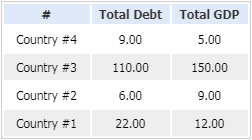# Debt-to-GDP Ratio Calculator

This Debt-to-GDP ratio calculator can be used for calculating the ratio between a nation's debt and its GDP (gross domestic product).

How to use the calculator: Input the relevant information into the form below and click on the "Calculate Debt-to-GDP Ratio" button.

Debt to GDP Ratio Calculator

Results
Debt-to-GDP Ratio:
Debt-to-GDP Ratio =
=
=
= %

## Explaining the Debt-to-GDP Ratio

A frequently used economic measure, the debt/GDP ratio measures the level of debt a country has against its gross domestic product (GDP). This ratio is generally rendered as a percentage, and is a good indicator of how able the country is to repay its debts. Put another way, debt-to-GDP ratios measure public debt against yearly economic output. The ratio can demonstrate how long a nation would need to repay all its debts, if all of its GDP was used for the purpose.

Countries that can maintain interest payments on their debts, without needing to refinance or adversely affect economic growth, are usually regarded as stable. Countries that have high debt-to-GDP ratios generally find it difficult to pay off external debts (also referred to as "public debts"), these being any monies owing to external lenders. Such nations are frequently faced with demands for high interest rates on any money they borrowed. If a country has a very high debt-to-GDP ratio, they may find it difficult to borrow money at all.

## Debt-to-GDP Ratio Formula

We can calculate the ratio using the following formula:

Debt-to-GDP Ratio (%) = (Total Debt of Country / Total GDP of Country) × 100

In this formula, Total Debt of Country represents the full amount of money owed by the national government, and Total GDP (Gross Domestic Product) of Country represents the value of all goods and services the country's economy has produced in a particular year.

## Example

Let's take a look at four imaginary countries and their national debts and gross domestic product for 2020 (in billion dollars):Using the formula described above, we can calculate the debt-to-GDP ratio for every country, thus:

Country #1:  \$22 / \$12 = 183.33%

Country #2:  \$6 / \$9 = 66.67%

Country #3:  \$110 / \$150 = 73.33%

Country #4:  \$9 / \$5 = 180.00%

By making a calculation of the debt-to-GDP ratio, it is clear that the first and fourth countries have the highest ratios. The higher this ratio is, the more likely it is that the country won't be able to repay its debts. Ultimately this could mean that the country might default on its debt, causing financial panic both domestically and internationally.